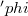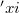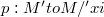﻿

### Symmetries which preserve the characteristic vector field of K-contact manifolds

#### Abstract

As is well-known locally symmetric K-contact manifolds are spaces of constant curvature ().This means that having isometric local geodesic symmetries is a very strong restriction for K-contact manifolds.Thus other classes of isometries shall fit for contact geometry. For example, T. Takahashi  has introduced the notion of$'phi$-geodesic symmetries on Sasaki manifolds and also on K-contact manifolds. Since then, manifolds with such isometries have been studied extensively.In this paper we generalize the notion of$'phi$-geodesic symmetries. Because we notice that our diffeomorphisms preserve the characteristic vector field$'xi$ of K-contact manifolds, we call them symmetries which preserve the characteristic vector-field, or$'xi$-preserving symmetries.Our idea for a construction of such local diffeomorphisms on K-contact manifolds is the lifting of symmetries on almost Kähler manifolds through the local fibering$p : M'to M/'xi$ of K-contact manifolds.After recalling elementary facts on contact geometry in Section 1, we devote Section 2 to our definition of symmetries which preserve the characteristic vector field.Also we construct such a family of symmetries, which is an example of local S-rotations around curves in the sense of L. Nicolodi and L. Vanhecke .In Section 3 we give some examples of our symmetries.

DOI Code: 10.1285/i15900932v13n2p229

Full Text: PDF